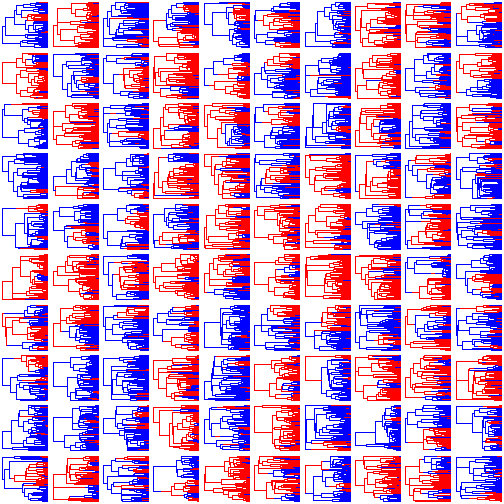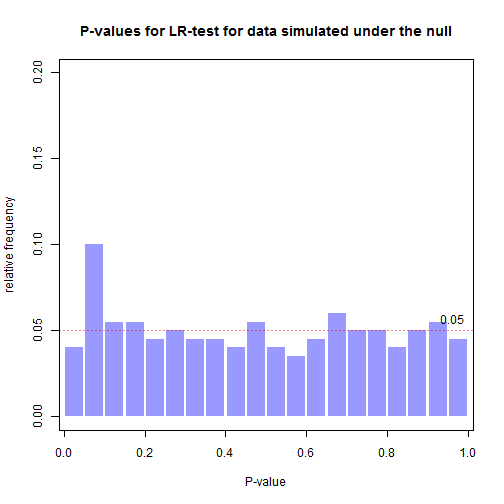## Tuesday, December 5, 2017

### Type I error rates for variable-rate/process Mk discrete character evolution model

Earlier today I described a new method for testing hypotheses about heterogeneity in the rate (or process) of discrete character evolution across a tree.

Although mathematically quite different, this methods owes its intellectual provenance to an article by Brian O'Meara & colleagues published nearly a dozen years ago in 2006. The tactic here is basically the same, but applied to discrete rather than continuous traits.

I just pushed another small update that modifies the `logLik` methods of the `"fitMk"` and `"fitmultiMk"` object classes to facilitate comparison of models in which the rate (or process) is either homogeneous (`"fitMk"`) or non-homogeneous (`"fitmultiMk"`) across the edges of the tree.

In ensuring too that full credit goes where it is due, I should note that for computing the likelihood I adapted Felsenstein's pruning algorithm as implemented in Emmanuel Paradis's ape package.

Below, I'll examine the type I error of the method when used in hypothesis testing against a homogeneous rate/process model.

First, we need to simulate our 'regimes' on the trees. These could be specified arbitrarily, but in this case I will just simulate a binary character on a set of pure-birth phylogenies using `sim.history`:

``````library(phytools)
packageVersion("phytools")
``````
``````##  '0.6.51'
``````
``````trees<-pbtree(n=100,scale=1,nsim=200)
Q<-matrix(c(-0.5,0.5,0.5,-0.5),2,2)
rownames(Q)<-colnames(Q)<-letters[1:2]
trees<-lapply(trees,sim.history,Q=Q,message=FALSE)
class(trees)<-c("multiSimmap","multiPhylo")
``````

I generated 200 trees. Let's plot the first 100 of these:

``````par(mfrow=c(10,10))
nulo<-sapply(trees[1:100],plot,colors=setNames(c("blue","red"),
letters[1:2]),ftype="off",lwd=1)
``````Next, we can simulate a discrete character on each of these trees, but in which the transition process is unrelated to the mapped regimes - that is, in which the null hypothesis of no difference in rate or process exists:

``````Q<-matrix(c(-1,1,1,-1),2,2)
rownames(Q)<-colnames(Q)<-0:1
X<-lapply(trees,function(x,Q) getStates(sim.history(x,Q,
message=FALSE),"tips"),Q=Q)
``````

Now let's fit our two models to each data vector & tree:

``````fits.single<-mapply(fitMk,tree=trees,x=X,MoreArgs=list(model="ER"),
SIMPLIFY=FALSE)
fits.multi<-mapply(fitmultiMk,tree=trees,x=X,MoreArgs=list(model="ER"),
SIMPLIFY=FALSE)
``````

We can look at a single fitted model of each type:

``````fits.single[]
``````
``````## Object of class "fitMk".
##
## Fitted (or set) value of Q:
##           0         1
## 0 -0.912078  0.912078
## 1  0.912078 -0.912078
##
## Fitted (or set) value of pi:
##   0   1
## 0.5 0.5
##
## Log-likelihood: -41.127165
##
## Optimization method used was "nlminb"
``````
``````fits.multi[]
``````
``````## Object of class "fitmultiMk".
##
## Fitted value of Q[a]:
##           0         1
## 0 -0.888472  0.888472
## 1  0.888472 -0.888472
##
## Fitted value of Q[b]:
##           0         1
## 0 -1.032995  1.032995
## 1  1.032995 -1.032995
##
## Fitted (or set) value of pi:
##   0   1
## 0.5 0.5
##
## Log-likelihood: -41.116092
##
## Optimization method used was "nlminb"
``````

We can also conduct a likelihood-ratio test on each pair of fitted models. I will do that using `lmtest::lrtest` as follows:

``````library(lmtest)
suppressWarnings(LR.test<-mapply(lrtest,fits.single,fits.multi,
SIMPLIFY=FALSE))
## for example
LR.test[]
``````
``````## Likelihood ratio test
##
## Model 1: dots[[1L]][[1L]]
## Model 2: dots[[2L]][[1L]]
##   #Df  LogLik Df  Chisq Pr(>Chisq)
## 1   1 -41.127
## 2   2 -41.116  1 0.0221     0.8817
``````

Let's pull out the P-values from these tests & plot them:

``````P<-sapply(LR.test,function(x) x[["Pr(>Chisq)"]])
obj<-hist(P,20,plot=FALSE)
plot(obj\$mids,obj\$counts/sum(obj\$counts),type="h",
lwd=18,col=make.transparent("blue",0.4),lend=1,
xlab="P-value",ylab="relative frequency",ylim=c(0,0.2))
title(main="P-values for LR-test for data simulated under the null")
abline(h=0.05,col=make.transparent("red",0.5),lwd=1,
lty="dotted")
text(0.96,0.05,"0.05",pos=3)
``````Neat. This is close to what we'd hope for.